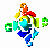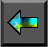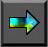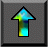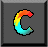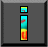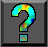Next: Chapter Notes Up: 5 Compositional C++ Previous: 5.13 Summary

# Exercises

1. Extend Program 5.4 to allow for nonbinary trees: that is, trees with an arbitrary number of subtrees rooted at each node.

2.   Design and construct a CC++ implementation of the manager/worker structure used in the parameter study problem described in Section 1.4.4.

3. Design and construct a decentralized version of the manager/worker structure developed in Exercise 2. Design and carry out experiments to determine when each version is more efficient.

4. Design and implement a program that can be used to quantify CC++ processor object and thread creation costs, both within the same processor and on remote processors. Conduct experiments to measure these costs, and obtain estimates for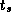and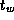.

5.   Implement and instrument the channel library presented in Section 5.11, and use this code to measure CC++ communication costs on various parallel computers.

6. Modify the program developed in Exercise 5 to use spawn to implement the RPC used for a send operation, and conduct experiments to compare the performance of the two versions.

7.   Complete Program 5.13, using the channel library of Section 5.11 to perform communication.

8.   Complete Program 5.14, using the channel library of Section 5.11 to perform communication.

9. Design and carry out experiments to compare the performance of the programs developed in Exercises 7 and 8.

10. Use the POArray class of Program 5.10 to implement a version of Program 5.4 in which search tasks are implemented as threads mapped to a fixed number of processor objects.

11. Extend the program developed in Exercise 7 to provide a 2-D decomposition of principal data structures.

12. Extend the channel library presented in Section 5.11 to allow polling for pending messages.

13. Extend the channel library presented in Section 5.11 to provide a merger that allows multiple senders on a channel.

14. Implement a hypercube communication template (see Chapter 11). Use this template to implement simple reduction, vector reduction, and broadcast algorithms.

15. Construct a CC++ implementation of the tuple space module described in Section 4.5. Use this module to implement the database search problem described in that section.Next: Chapter Notes Up: 5 Compositional C++ Previous: 5.13 Summary